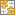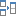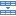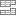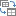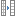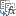# Excel FILTER function with formula examples

In this quick lesson, you will learn how to filter in Excel dynamically with formulas. Examples to filter duplicates, cells containing certain text, with multiple criteria, and more.

How do you usually filter in Excel? For the most part, by using Auto Filter, and in more complex scenarios with Advanced Filter. Being fast and powerful, these methods have one significant drawback - they do not update automatically when your data changes, meaning you would have to clean up and filter again. The introduction of the FILTER function in Excel 365 becomes a long-awaited alternative to the conventional features. Unlike them, Excel formulas recalculate automatically with each worksheet change, so you'll need to set up your filter just once!

## Excel FILTER function

The FILTER function in Excel is used to filter a range of data based on the criteria that you specify.

The function belongs to the category of Dynamic Arrays functions. The result is an array of values that automatically spills into a range of cells, starting from the cell where you enter a formula.

The syntax of the FILTER function is as follows:

FILTER(array, include, [if_empty])

Where:

• Array (required) - the range or array of values that you want to filter.
• Include (required) - the criteria supplied as a Boolean array (TRUE and FALSE values).
Its height (when data is in columns) or width (when data is in rows) must be equal to the that of the array argument.
• If_empty (optional) - the value to return when no entries meet the criteria.
Note. Currently the FILTER function is only available with Office 365 subscriptions. In Excel 2019, Excel 2016 and earlier versions, it is not supported.

## Basic Excel FILTER formula

For starters, let's discuss a couple of very simple cases just to gain more understanding how an Excel formula to filter data works.

From the below data set, supposing you want to extract the records with a specific value in the Group, column, say group C. To have it done, we supply the expression B2:B13="C" to the include argument, which will produce a required Boolean array, with TRUE corresponding to "C" values.

`=FILTER(A2:C13, B2:B13="C", "No results")`

In practice, it's more convenient to input the criteria in a separate cell, e.g. F1, and use a cell reference instead of hardcoding the value directly in the formula:

`=FILTER(A2:C13, B2:B13=F1, "No results")`

Unlike Excel's Filter feature, the function does not make any changes to the original data. It extracts the filtered records into the so-called spill range (E4:G7 in the screenshot below), beginning in the cell where the formula is entered: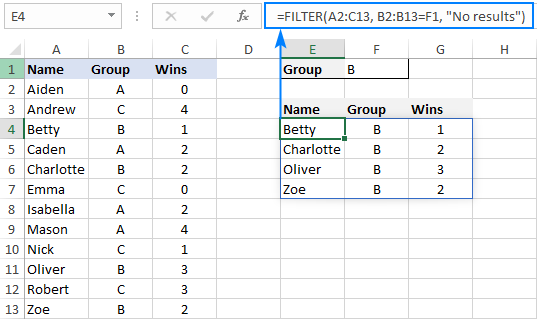If no records match the specified criteria, the formula returns the value you put in the if_empty argument, "No results" in this example: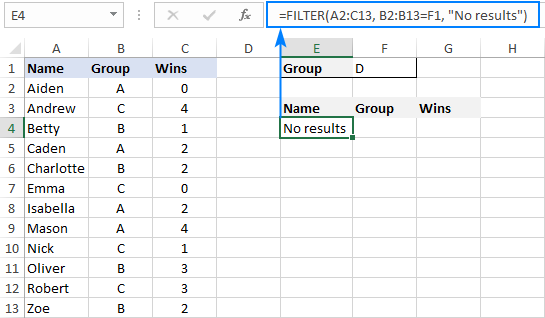If you'd rather return nothing in this case, then supply an empty string ("") for the last argument:

`=FILTER(A2:C13, B2:B13=F1, "")`

In case your data is organized horizontally from left to right like shown in the screenshot below, the FILTER function will work nicely too. Just make sure you define appropriate ranges for the array and include arguments, so that the source array and Boolean array have the same width:

`=FILTER(B2:M4, B3:M3= B7, "No results")`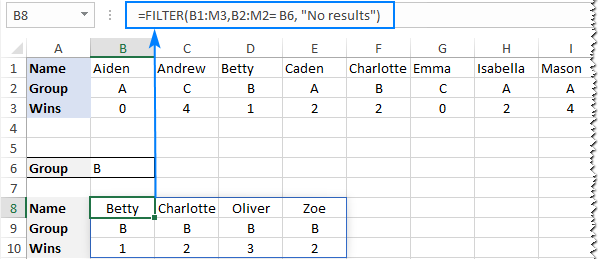### Excel FILTER function - usage notes

To effectively filter in Excel with formulas, here are a couple of important points to take notice of:

• The FILTER function automatically spills the results vertically or horizontally in the worksheet, depending on how your original data is organized. So, please make sure you always have enough empty cells down and to the right, otherwise you'll get a #SPILL error.
• The results of the Excel FILTER function are dynamic, meaning they update automatically when values in the original data set change. However, the range supplied for the array argument is not updated when new entries are added to the source data. If you wish the array to resize automatically, then convert it to an Excel table and build formulas with structured references, or create a dynamic named range.

## How to filter in Excel - formula examples

Now that you know how a basic Excel filter formula works, it's time to get some insights into how it could be extended for solving more complex tasks.

### Filter with multiple criteria (AND logic)

To filter data with multiple criteria, you supply two or more logical expressions for the include argument:

The multiplication operation processes the arrays with the AND logic, ensuring that only the records that meet all the criteria are returned. Technically, it works this way:

The result of each logical expression is an array of Boolean values, where TRUE equates to 1 and FALSE to 0. Then, the elements of all the arrays in the same positions are multiplied. Since multiplying by zero always gives zero, only the items for which all the criteria are TRUE get into the resulting array, and consequently only those items are extracted.

The below examples show this generic formula in action.

#### Example 1. Filter multiple columns in Excel

Extending our basic Excel FILTER formula a little further, let's filter the data by two columns: Group (column B) and Wins (column C).

For this, we set up the following criteria: type the name of the target group in F2 (criteria1) and the minimum required number of wins in F3 (criteria2).

Given that our source data is in A2:C13 (array), groups are in B2:B13 (range1) and wins are in C2:C13 (range2), the formula takes this form:

`=FILTER(A2:C13, (B2:B13=F2) * (C2:C13>=F3), "No results")`

As the result, you get a list of players in group A who have secured 2 or more wins: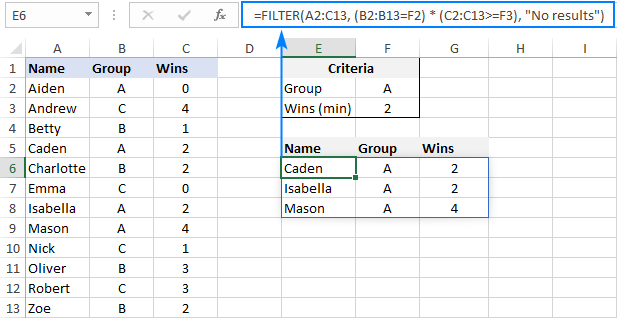#### Example 2. Filter data between dates

First off, it should be noted that it's not possible to make up a generic formula to filter by date in Excel. In different situations, you will need to build criteria differently, depending on whether you want to filter by a specific date, by month, or by year. The purpose of this example is to demonstrate the general approach.

To our sample data, we add one more column containing the dates of the last win (column D). And now, we will extract the wins that occurred in a specific period, say between May 17 and May 31.

Please notice that in this case, both criteria apply to the same range:

`=FILTER(A2:D13, (D2:D13>=G2) * (D2:D13<=G3), "No results")`

Where G2 and G3 are the dates to filter between.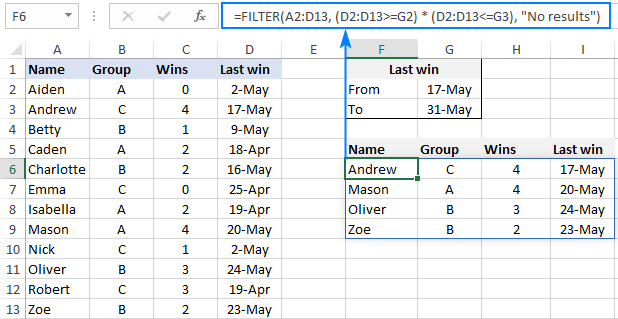### Filter with multiple criteria (OR logic)

To extract data based on multiple OR condition, you also use logical expressions like shown in the previous examples, but instead of multiplying, you add them up. When the Boolean arrays returned by the expressions are summed, the resulting array will have 0 for entries that do not meet any criteria (i.e. all the criteria are FALSE), and such entries will be filtered out. The entries for which at least one criterion is TRUE will be extracted.

Here's the generic formula to filter columns with the OR logic:

As an example, let's extract a list of players that have this or that number of wins.

Assuming the source data is in A2:C13, wins are in C2:C13, and the win numbers of interest are in F2 and F3, the formula would go as follows:

`=FILTER(A2:C13, (C2:C13=F2) + (C2:C13=F3), "No results")`

As the result, you know which players have won all the games (4) and which have won none (0):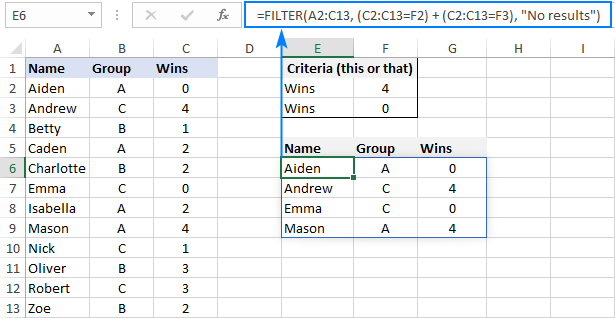### Filter based on multiple AND as well as OR criteria

In situation when you need to apply both criteria types, remember this simple rule: join the AND criteria with asterisk (*) and OR criteria with the plus sign (+).

For example, to return a list of players that have a given number of wins (F2) AND belong to the group mentioned in either E2 OR E3, build the following chain of logical expressions:

`=FILTER(A2:C13, (C2:C13=F2) * ((B2:B13=E2) + (B2:B13=E3)), "No results")`

And you will get the following result: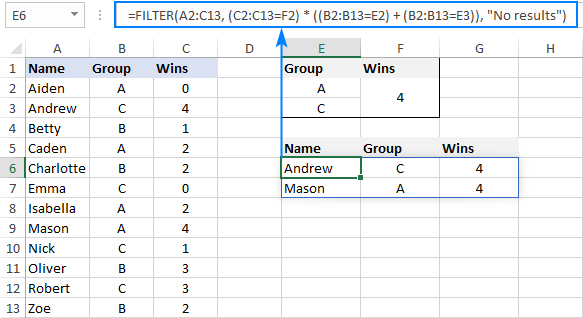### How to filter duplicates in Excel

When working with huge worksheets or combining data from different sources, there's often a possibility that some duplicates would sneak in.

If you are looking to filter out duplicates and extract unique items, then use the UNIQUE function as explained in the above linked tutorial.

If your goal is to filter duplicates, i.e. extract entries that occur more than once, then use the FILTER function together with COUNTIFS.

The idea is to get the occurrences counts for all the records and extract those greater than 1. To get the counts, you supply the same range for each criteria_range / criteria pair of COUNTIFS like this:

For example, to filter duplicate rows from the data in A2:C20 based on the values in all 3 columns, here's the formula to use:

`=FILTER(A2:C20, COUNTIFS(A2:A20, A2:A20, B2:B20, B2:B20, C2:C20, C2:C20)>1, "No results")`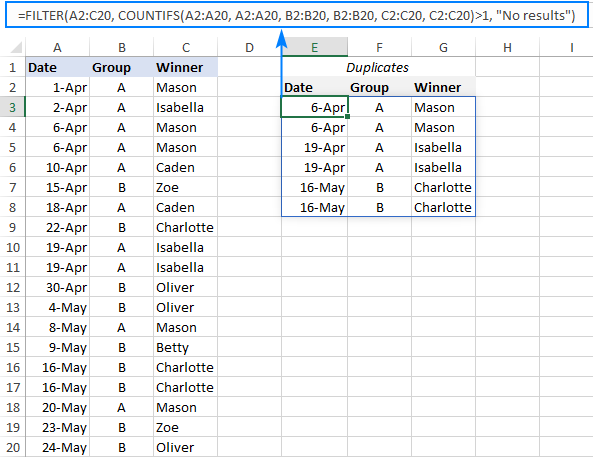Tip. To filter duplicates based on the values in the key columns, include only those columns in the COUNTIFS function.

### How to filter out blanks in Excel

A formula for filtering out blank cells is, in fact, a variation of the Excel FILTER formula with multiple AND criteria. In this case, we check whether all (or particular) columns have any data in them and exclude the rows where at least one cell is empty. To identify non-blank cells, you use the "not equal to" operator (<>) together with an empty string ("") like this:

With the source data in A2:C12, to filter out rows containing one or more blank cells, the following formula is entered in E3: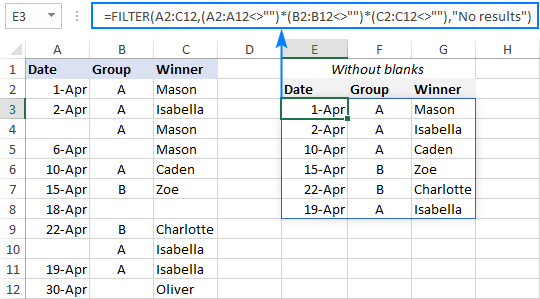### Filter cells containing specific text

To extract cells that contain certain text, you can use the FILTER function together with the classic If cell contains formula:

Here's how it works:

• The SEARCH function looks for a specified text string in a given range and returns either a number (the position of the first character) or #VALUE! error (text not found).
• The ISNUMBER function converts all the numbers to TRUE and errors to FALSE and passes the resulting Boolean array to the include argument of the FILTER function.

For this example, we've added the Last names of players in B2:B13, typed the part of the name we want to find in G2, and then use the following formula to filter the data:

`=FILTER(A2:D13, ISNUMBER(SEARCH(G2, B2:B13)), "No results")`

As the result, the formula retrieves the two surnames containing "han":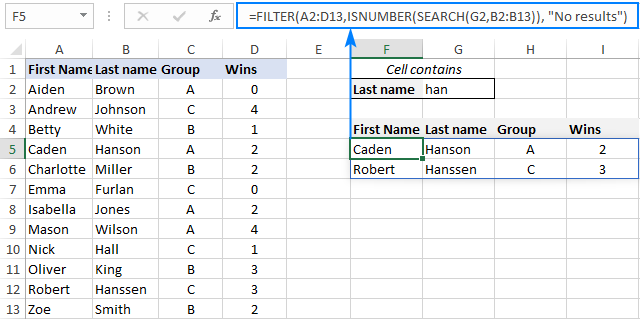### Filter and calculate (Sum, Average, Min, Max, etc.)

A cool thing about the Excel FILTER function is that it can not only extract values with conditions, but also summarize the filtered data. For this, combine FILTER with aggregation functions such as SUM, AVERAGE, COUNT, MAX or MIN.

For instance, to aggregate data for a specific group in F1, use the following formulas:

Total wins:

`=SUM(FILTER(C2:C13, B2:B13=F1, 0))`

Average wins:

`=AVERAGE(FILTER(C2:C13, B2:B13=F1, 0))`

Maximum wins:

`=MAX(FILTER(C2:C13, B2:B13=F1, 0))`

Minimum wins:

`=MIN(FILTER(C2:C13, B2:B13=F1, 0))`

Please pay attention that, in all the formulas, we use zero for the if_empty argument, so the formulas would return 0 if no values meeting the criteria are found. Supplying any text such as “No results” would result in a #VALUE error, which is obviously the last thing you want :)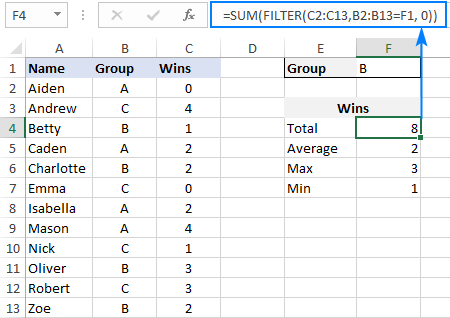### Case-sensitive FILTER formula

A standard Excel FILTER formula is case-insensitive, meaning it makes no distinction between lowercase and uppercase characters. To distinguish text case, nest the EXACT function in the include argument. This will force FILTER to do logical test in a case-sensitive manner:

Supposing, you have both groups A and a and wish to extract records where the group is the lowercase "a". To have it done, use the following formula, where A2:C13 is the source data and B2:B13 are groups to filter:

`=FILTER(A2:C13, EXACT(B2:B13, "a"), "No results")`

As usual, you can input the target group in a predefined cell, say F1, and use that cell reference instead of hardcoded text:

`=FILTER(A2:C13, EXACT(B2:B13, F1), "No results")`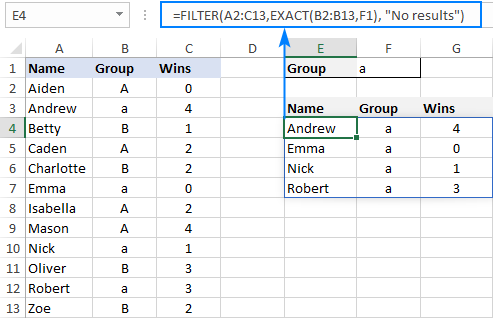## How to FILTER data and return only specific columns

For the most part, filtering all columns with a single formula is what Excel users want. But if your source table contains tens or even hundreds of columns, you may certainly want to limit the results to a few most important ones.

### Example 1. Filter some adjacent columns

In situation when you want some neighboring columns to appear in a FILTER result, include only those columns in array because it is this argument that determines which columns to return.

In the basic FILTER formula example, supposing you wish to return the first 2 columns (Name and Group). So, you supply A2:B13 for the array argument:

`=FILTER(A2:B13, B2:B13=F1, "No results")`

As the result, we get a list of participants of the target group defined in F1: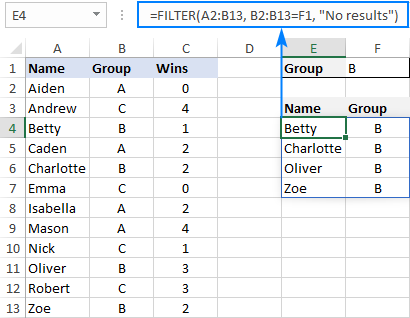### Example 2. Filter non-adjacent columns

To cause the FILTER function to return non-contiguous columns, use this clever trick:

1. Make a FILTER formula with the desired condition(s) using the entire table for array.
2. Nest the above formula inside another FILTER function. To configure the "wrapper" function, use an array constant of TRUE and FALSE values or 1's and 0's for the include argument, where TRUE (1) represents the columns to be kept and FALSE (0) marks the columns to be excluded.

For example, to return only Names (1st column) and Wins (3rd column), we are using {1,0,1} or {TRUE,FALSE,TRUE} for the include argument of the outer FILTER function:

`=FILTER(FILTER(A2:C13, B2:B13=F1), {1,0,1})`

Or

`=FILTER(FILTER(A2:C13, B2:B13=F1), {TRUE,FALSE,TRUE})`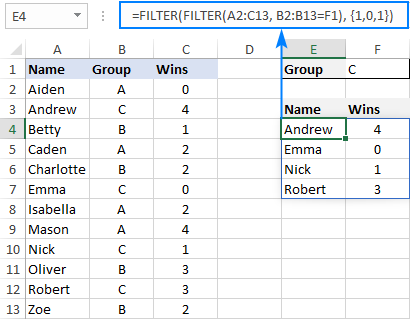## Excel FILTER function not working

In situation when your Excel FILTER formula results in an error, most likely that will be one of the following:

### #CALC! error

Occurs if the optional if_empty argument is omitted, and no results meeting the criteria are found. The reason is that currently Excel does not support empty arrays. To prevent such errors, be sure to always define the if_empty value in your formulas.

### #VALUE error

Occurs when the array and include argument have incompatible dimensions.

### #N/A, #VALUE, etc.

Different errors may occur if some value in the include argument is an error or cannot be converted to a Boolean value.

### #NAME error

Occurs when trying to use FILTER in an older version of Excel. Please remember that it is a new function, which is only available in Office 365 subscriptions.

In Excel 365, a #NAME error occurs if you accidentally misspell the function's name.

### #SPILL error

Occurs if one or more cells in the spill range are not completely blank. To fix it, just clear or delete obstructing non-empty cells.

### #REF! error

Occurs when a FILTER formula is used between different workbooks, and the source workbook is closed.

That's how to filer data in Excel dynamically. I thank you for reading and hope to see you on our blog next week!

Filter in Excel with formulas (.xlsx file)

### 16 responses to "Excel FILTER function with formula examples"

1.Lincoln Oliver says:

Hello!

One doubt:

How do I Add a last row with column sum, using filter? Could I do that?

•Svetlana Cheusheva (Ablebits.com Team) says:

Hi Lincoln,

This can be done by using the SUM function with a spill range reference (# symbol).

Assuming the FILTER formula is in E4, the SUM formula will be as simple as this:
=SUM(E4#)

Just make sure there are enough empty rows between the FILTER and SUM formula cells. If FILTER does not have the required number of rows to fill with the results, a #SPILL error will occur.

2.Matt says:

Hi,

In your examples, how do you return just Name and Wins for example

3.Manuel Isaías says:

Is it posible to filter if a column matches any of a list of values.

Example:

List of criterias
A1: 1
A2: 2
A3: 3
A4: 4

The data i want to filter:
B1: 1
B2: 4
B3: 3
B4: 6
B5: 7
B6: 2

Result:
1
4
3
2

is there a way to do this?

4.Tripp says:

With is formula I pull all rows for the week and corresponding times. I sort on the week. Is it possible to add a blank line to separate each day of the week? My spreadsheet has 85 columns, I show column 1 (date) and column 2 (key time). I also need sort both the Date and Key Time. Thank you for any assistance.

=FILTER(SORT(FILTER(TDaily[[Date]:[Key Time]], (TDaily[Date]>=I10) * (TDaily[Date]<=I11)), 1, 1), {1,0,0,0,0,0,0,0,0,0,0,0,0,0,0,0,0,0,0,0,0,0,0,0,0,0,0,0,0,0,0,0,0,0,0,0,0,0,0,0,0,0,0,0,0,0,0,0,0,0,0,0,0,0,0,0,0,0,0,0,0,0,0,0,0,0,0,0,0,0,0,0,0,0,0,0,0,0,0,0,0,0,82})

•Alexander Trifuntov (Ablebits.com Team) says:

Hello!
The FILTER function returns an array of values. Therefore, it cannot return any empty lines. If you create a pivot table from this data, then different formatting methods are possible there.

5.Jim says:

How do i create a filter on an ever changing range (sometimes 100 rows, sometimes 250, or even 10) - do i have to preset the filter to cover x number of rows, or can i create dynamic range

6.Cameron Stewart says:

Regarding using aggregate functions wrapped around filter results ... set {is empty] to zero works fine for most aggregate functions such as SUM, but is problematic for COUNT functions. The intention behind wrapping the filter function with COUNT is to count rows. If no rows exist matching the filter criteria, then , I want the COUNT function to return zero. Maybe I am missing something, but this is a lot harder to do than I thought. Maybe I need to abandon the FILTER and UNIQUE function to use other methods. Any suggestions? Thanks

•Alexander Trifuntov (Ablebits.com Team) says:

Hello!
You have not listed the formulas that are causing the problem. But I think using function IFERROR will help replace the error message with zero.

7.Ram G says:

Dear Sir,how to filter data ,(Like Company Record of Daily Expanse to Employees,i want to select only one date and the all associated data of that day will show by choosing date)

•Alexander Trifuntov (Ablebits.com Team) says:

Hello!
If the required date is written in a cell (for example, F1), then you can use the formulas from the second section of this article. If you want to write a date into a formula, use the DATE function.

8.Swapnesh says:

I'm trying to enter this function into an xlsm sheet whic has other macros. However, it is returning error #Value... any help would be really appreciated

Im unable to upload my file or could have shared the same as well

•Alexander Trifuntov (Ablebits.com Team) says:

Hello!
Check the data in the cells you are referencing. Do they match the value in the filter?
Please specify, what formula you used. Give an example of the source data.
It’ll help me understand your request better and find a solution for you.